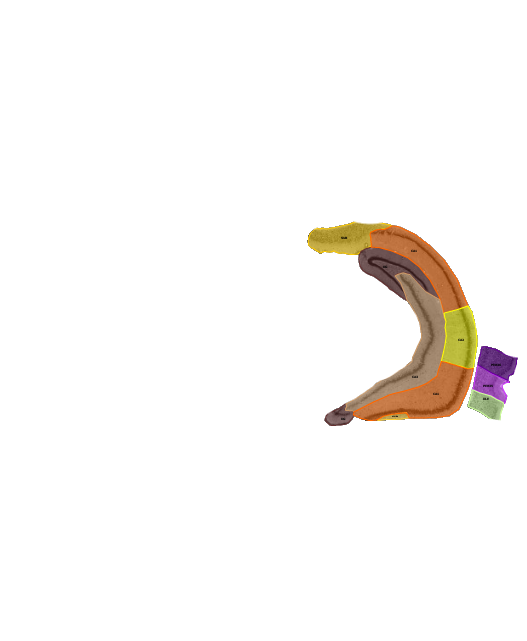# Masking with Matlab

Recently, I had a bunch of images for which I wanted to make all grey pixels transparent, in order to just see the color coded regions.This was one of the situations, in which I was wondering whether I should do it manually (for 37 images) with Gimp, or write a script that can do the job for me. I was happy, that I decided to write a script, because it turned out that it just took me one minute to figure out the commands in Matlab and an additional minute to write the script. At the end I saved a lot of time and obtained images with an even more accurate mask than those images that I produced with Gimp. Here is the script for this kind of job:

``` function maskImage(filename) a = imread(filename); c = ((a(:,:,1) == a(:,:,2)).* (a(:,:,2) == a(:,:,3))) == 0; imwrite(a, filename, 'png', 'Alpha', double(c));```